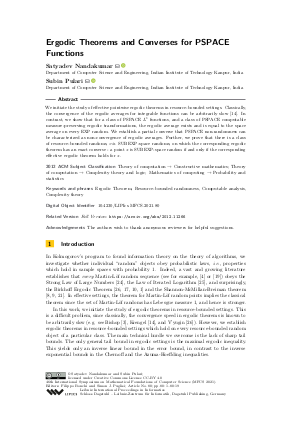Document# Ergodic Theorems and Converses for PSPACE Functions

### Authors Satyadev Nandakumar, Subin Pulari## File

LIPIcs.MFCS.2021.80.pdf
• Filesize: 0.78 MB
• 19 pages

## Acknowledgements

The authors wish to thank anonymous reviewers for helpful suggestions.

## Cite As

Satyadev Nandakumar and Subin Pulari. Ergodic Theorems and Converses for PSPACE Functions. In 46th International Symposium on Mathematical Foundations of Computer Science (MFCS 2021). Leibniz International Proceedings in Informatics (LIPIcs), Volume 202, pp. 80:1-80:19, Schloss Dagstuhl - Leibniz-Zentrum für Informatik (2021)
https://doi.org/10.4230/LIPIcs.MFCS.2021.80

## Abstract

We initiate the study of effective pointwise ergodic theorems in resource-bounded settings. Classically, the convergence of the ergodic averages for integrable functions can be arbitrarily slow [Ulrich Krengel, 1978]. In contrast, we show that for a class of PSPACE L¹ functions, and a class of PSPACE computable measure-preserving ergodic transformations, the ergodic average exists and is equal to the space average on every EXP random. We establish a partial converse that PSPACE non-randomness can be characterized as non-convergence of ergodic averages. Further, we prove that there is a class of resource-bounded randoms, viz. SUBEXP-space randoms, on which the corresponding ergodic theorem has an exact converse - a point x is SUBEXP-space random if and only if the corresponding effective ergodic theorem holds for x.

## Subject Classification

##### ACM Subject Classification
• Theory of computation → Constructive mathematics
• Theory of computation → Complexity theory and logic
• Mathematics of computing → Probability and statistics
##### Keywords
• Ergodic Theorem
• Resource-bounded randomness
• Computable analysis
• Complexity theory

## Metrics

• Access Statistics
• Total Accesses (updated on a weekly basis)
0

## References

1. L. Bienvenu, A. Day, M. Hoyrup, I. Mezhirov, and A. Shen. A constructive version of Birkhoff’s ergodic theorem for Martin-Löf random points. Information and Computation, 210:21-30, 2012.2. P. Billingsley. Probability and Measure, second edition. John Wiley and Sons, New York, N.Y., 1986.3. E. Bishop. Foundations of constructive analysis. McGraw-Hill, New York, 1967.4. Rodney G Downey and Denis R Hirschfeldt. Algorithmic randomness and complexity. Springer Science & Business Media, 2010.5. Johanna NY Franklin and Henry Towsner. Randomness and non-ergodic systems. Moscow Mathematical Journal, 14:711-744, 2014.6. Stefano Galatolo, Mathieu Hoyrup, and Cristóbal Rojas. A constructive Borel-Cantelli lemma. constructing orbits with required statistical properties. Theoretical Computer Science, 410(21-23):2207-2222, 2009.7. S. Galotolo, M. Hoyrup, and C. Rojas. Computing the speed of convergence of ergodic averages and pseudorandom points in computable dynamical systems. In 7th International Conference on Computability and Complexity in Analysis, pages 7-18, 2010.8. Michael Hochman. Upcrossing ineualities for stationary sequences and applications. Annals of Probability, 37(6):2135-2149, 2009.9. Mathieu Hoyrup. The dimension of ergodic random sequences. In Proceedings of the Symposium on Theoretical Aspects of Computer Science, pages 567-576, 2012.10. Mathieu Hoyrup and Cristóbal Rojas. Applications of effective probability theory to Martin-Löf randomness. In International Colloquium on Automata, Languages, and Programming, pages 549-561. Springer, 2009.11. Mathieu Hoyrup and Cristóbal Rojas. Computability of probability measures and martin-löf randomness over metric spaces. Information and Computation, 207(7):830-847, 2009.12. Xiang Huang and D. M. Stull. Polynomial space randomness in analysis. In MFCS, 2016.13. Ker-I Ko. Complexity theory of real functions. Springer Science & Business Media, 2012.14. Ulrich Krengel. On the speed of convergence in the ergodic theorem. Monatshefte für Mathematik, 86:3-6, 1978.15. L. Kuipers and H. Niederreiter. Uniform distribution of sequences. Wiley-Interscience [John Wiley & Sons], New York-London-Sydney, 1974. Pure and Applied Mathematics.16. John E. Maxfield. A short proof of Pillai’s theorem on normal numbers. Pacific Journal of Mathematics, 2(1):23-24, 1952.17. S. Nandakumar. An effective ergodic theorem and some applications. In Proceedings of the 40th Annual Symposium on the Theory of Computing, pages 39-44, 2008.18. Satyadev Nandakumar, Subin Pulari, Prateek Vishnoi, and Gopal Viswanathan. An analogue of pillai’s theorem for continued fraction normality and an application to subsequences. arXiv preprint, 2019. URL: http://arxiv.org/abs/1909.03431.
19. André Nies. Computability and randomness, volume 51. OUP Oxford, 2009.20. S. S. Pillai. On normal numbers. Proceedings of the Indian Academy of Sciences Sect. A, 11:73-80, 1940.21. Jason Rute. Topics in algorithmic randomness and computable analysis. PhD thesis, Carnegie Mellon University, August 2013.22. Donald M Stull. Algorithmic randomness and analysis. PhD thesis, Iowa State University, Ames, Iowa, USA, 2017.23. Donald M. Stull. Resource bounded randomness and its applications. Algorithmic Randomness: Progress and Prospects, 50:301, 2020.24. M. van Lambalgen. Random Sequences. PhD thesis, Department of Mathematics, University of Amsterdam, 1987.25. V. G. Vovk. The law of the iterated logarithm for random Kolmogorov, or chaotic, sequences. Theory of Probability and its Applications, 32(3):413-425, 1988.26. V. V. V'yugin. Effective convergence in probability and an ergodic theorem for individual random sequences. Theory of Probability and Its Applications, 42(1):39-50, 1997.X

Feedback for Dagstuhl Publishing